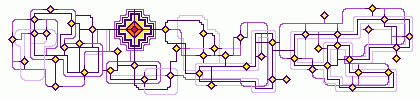## Network TheoryNature and the world of human technology are full of networks. People like to draw diagrams of networks: flow charts, electrical circuit diagrams, signal-flow graphs, Bayesian networks, Feynman diagrams and the like. Mathematically minded people know that in principle these diagrams fit into a common framework, namely category theory. But we are still far from a unified theory of networks. Here we explain how networks of various different kinds can be seen morphisms in various different categories, and show how these examples are connected by functors.

You can see the slides here.

For videos and slides covering other aspects of network theory, try: